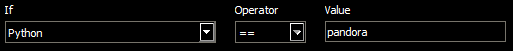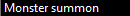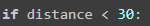# Spawn more pandora's box Plugin :D

If u need spawn more pandora’s box on ur spawn just use that simple plugin.
U can have only pandora in inventory! don’t use it with MSThat how u need set ur conditions for it
Also there is line where u set ur radius for this``````from phBot import *

pandorabox=2 #How many pandora's u need spawn?

def pandora():
p = get_position()
tp = get_training_area()
distance = round(GetDistance(p['x'], p['y'], tp['x'], tp['y']), 2)

if distance < 30:
k = get_monsters()
p = 0
for key in k:
if k[key]['name'] == "Pandora's Box":
p = p + 1
if p < pandorabox:
return True
return False

def GetDistance(ax, ay, bx, by):
return ((bx - ax) ** 2 + (by - ay) ** 2) ** 0.5